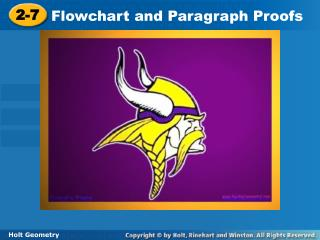Download Presentation2-72-7 - PowerPoint PPT Presentation

Download Presentation2-7
An Image/Link below is provided (as is) to download presentation

Download Policy: Content on the Website is provided to you AS IS for your information and personal use and may not be sold / licensed / shared on other websites without getting consent from its author. While downloading, if for some reason you are not able to download a presentation, the publisher may have deleted the file from their server.

- - - - - - - - - - - - - - - - - - - - - - - - - - - E N D - - - - - - - - - - - - - - - - - - - - - - - - - - -
Presentation Transcript

1. 2-7 Flowchart and Paragraph Proofs Holt Geometry

2. Warm Up Complete each sentence. 1.If the measures of two angles are ? , then the angles are congruent. 2. If two angles form a ? , then they are supplementary. 3. If two angles are complementary to the same angle, then the two angles are ? . equal linear pair congruent

3. Objectives Write flowchart and paragraph proofs. Prove geometric theorems by using deductive reasoning.

4. Vocabulary flowchart proof paragraph proof

5. A second style of proof is a flowchart proof, which uses boxes and arrows to show the structure of the proof. The justification for each step is written below the box.

6. Example 1: Reading a Flowchart Proof Use the given flowchart proof to write a two-column proof. Given: 2 and 3 are comp. 1  3 Prove: 2and 1are comp. Flowchart proof:

7. Check It Out! Example 1 Use the given flowchart proof to write a two-column proof. Given: RS = UV, ST = TU Prove: RT TV Flowchart proof:

8. Example 2: Writing a Flowchart Proof Use the given two-column proof to write a flowchart proof. Given: B is the midpoint of AC. Prove: 2AB = AC

9. Check It Out! Example 2 Use the given two-column proof to write a flowchart proof. Given: 2  4 Prove: m1  m3 Two-column Proof:

10. Check It Out! Example 2 Continued

11. A paragraph proof is a style of proof that presents the steps of the proof and their matching reasons as sentences in a paragraph. Although this style of proof is less formal than a two-column proof, you still must include every step.

12. Example 3: Reading a Paragraph Proof Use the given paragraph proof to write a two-column proof. Given: m1 + m2 = m4 Prove: m3 + m1 + m2 = 180° Paragraph Proof: It is given that m1 + m2 = m4. 3 and 4 are supplementary by the Linear Pair Theorem. So m3 + m4 = 180° by definition. By Substitution, m3 + m1 + m2 = 180°.

13. Lesson Quiz Use the two-column proof at right to write the following. 1. a flowchart proof 2. a paragraph proof